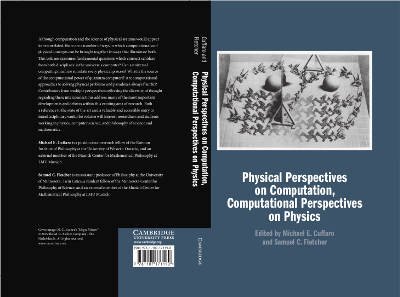## Janas, M., Cuffaro, M. E., and Janssen, M. Understanding Quantum Raffles: Quantum Mechanics on an Information-Theoretic Approach - Structure and Interpretation. Springer (series: Boston Studies in the Philosophy of Science), Forthcoming.

We use correlation arrays, the workhorse of Jeffrey Bub's Bananaworld, to analyze the correlations found in an experimental setup due to David Mermin for measurements on pairs of spin-1/2 particles in the singlet state. Adopting an approach pioneered by Itamar Pitowsky and promoted in Bananaworld, we geometrically represent the class of correlations allowed by quantum mechanics in this setup as an elliptope in a non-signaling cube, which represents the broader class of all correlations that cannot be used for the purpose of sending signals traveling faster than the speed of light. To determine which of these quantum correlations are allowed by so-called local hidden-variable theories, we investigate which ones we can simulate using raffles with baskets of tickets that have the outcomes for all combinations of measurement settings printed on them. The class of correlations found this way can be represented geometrically by a tetrahedron contained within the elliptope. We use the same Bub-Pitowsky framework to analyze a generalization of the Mermin setup for measurements on pairs of particles with higher spin in the singlet state. The class of correlations allowed by quantum mechanics in this case is still represented by the elliptope; the subclass of those whose main features can be simulated with our raffles can be represented by polyhedra that, with increasing spin, have more and more vertices and facets and get closer and closer to the elliptope. We use these results to advocate for Bubism (not to be confused with QBism), an interpretation of quantum mechanics along the lines of Bananaworld, belonging to the same lineage, or so we will argue, as the much-maligned Copenhagen interpretation. Probabilities and expectation values are primary in this interpretation. They are determined by inner products of state vectors in Hilbert space. State vectors do not themselves represent what is real in quantum mechanics. Instead the state vector gives a family of probability distributions over the values of subsets of observables, which do not add up to one overarching joint probability distribution over the values of all observables. As in classical theory, these values (along with the values of non-dynamical quantities such as charge or spin) represent what is real in the quantum world. Hilbert space puts constraints on possible combinations of such values, just as Minkowski space-time puts constraints on possible spatio-temporal constellations of events. Illustrating how generic such constraints are, the one derived in this volume, the equation for the elliptope, is a general constraint on correlation coefficients that can be found in older literature on statistics and probability theory. Udny Yule already stated the constraint. Bruno de Finetti already gave it a geometrical interpretation sharing important features with its interpretation in Hilbert space.

## Cuffaro, M. E. and Fletcher, S.C (eds.). Physical Perspectives on Computation, Computational Perspectives on Physics. Cambridge University Press (2018)Chapters: